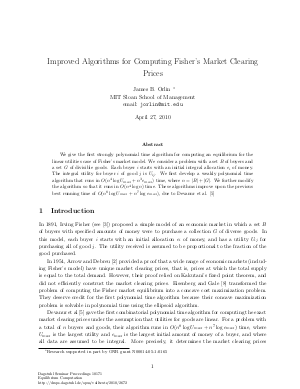Document# Improved Algorithms for Computing Fisher's Market Clearing Prices

### Author James B. Orlin## File

DagSemProc.10171.2.pdf
• Filesize: 245 kB
• 19 pages

## Cite As

James B. Orlin. Improved Algorithms for Computing Fisher's Market Clearing Prices. In Equilibrium Computation. Dagstuhl Seminar Proceedings, Volume 10171, pp. 1-19, Schloss Dagstuhl - Leibniz-Zentrum für Informatik (2010)
https://doi.org/10.4230/DagSemProc.10171.2

## Abstract

We give the first strongly polynomial time algorithm for computing an equilibrium for the linear utilities case of Fisher's market model. We consider a problem with a set \$B\$ of buyers and a set \$G\$ of divisible goods. Each buyer \$i\$ starts with an initial integral allocation \$e_i\$ of money. The integral utility for buyer \$i\$ of good \$j\$ is \$U_{ij}\$. We first develop a weakly polynomial time algorithm that runs in \$O(n^4 log U_{max} + n^3 e_{max})\$ time, where \$n = |B| + |G|\$. We further modify the algorithm so that it runs in \$O(n^4 log n)\$ time. These algorithms improve upon the previous best running time of \$O(n^8 log U_{max} + n^7 log e_{max})\$, due to Devanur et al.
##### Keywords
• Market equilibrium
• Fisher
• strongly polynomial

## Metrics

• Access Statistics
• Total Accesses (updated on a weekly basis)
0